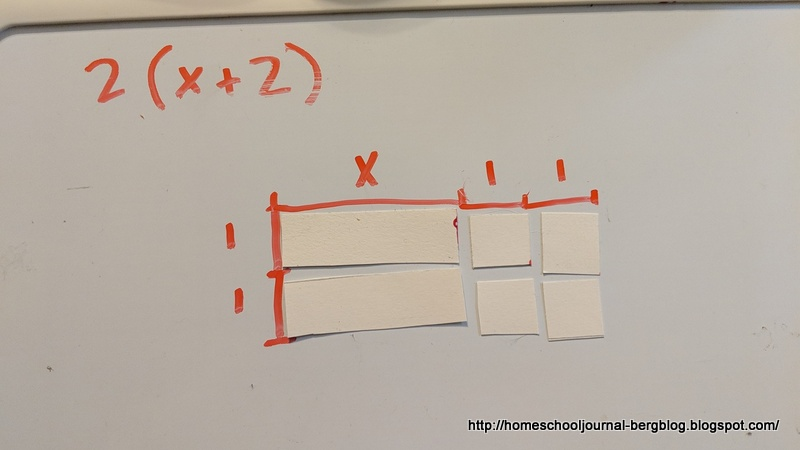Our Homeschool From Preschool to High School

Hands-on Algebra: The Distributive Property

We have used rectangle tiles to model multiplication. They can also be used to show the Distributive Property of Algebra.

Use a tile that is about 1 square unit. I am using about a 1-inch square of cardstock.

Next make an "x" tile by making a unit that is 1 unit wide and as long as you wish. For our purposes, we made it about three or four times as long, but remember that it is "x" units long.
We begin by using the tiles to find the product of 2 (x + 2)The rectangle has a width of 2 units and a length of x + 2 units. We can use our area tiles to mark off the dimensions on a mat, or in this case, a dry-erase board, that will show us the product. Using the marks as a guide, we make the rectangle with the algebra tiles.
The rectangle has 2 x-tiles and 4 1-tiles. The area of the rectangle is x + 1 + 1 + x + 1 + 1 or 2x + 4. Thus, 2(x + 2) = 2x + 4Now you just need to give your student some practice problems, (or he can even make up some of his own, if he's like.) He can use the tiles and a dry erase board and write the answers in his math journal or he could also solve problems in this way by sketching similar drawings in their math journals.
As a quiz, you could have your student write a paragraph explaining how to find the proof of such problems.